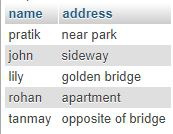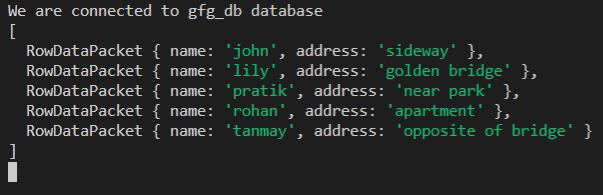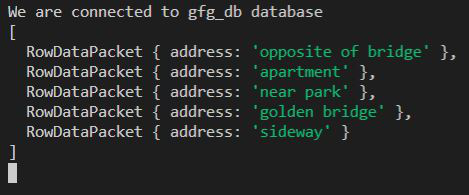Related Articles
NodeJS MySQL Order By Clause
• Difficulty Level : Medium
• Last Updated : 08 Feb, 2021

Introduction: We use the SQL ORDER BY Clause to sort the data with respect to some column value in ascending or descending order.

Syntax:

SELECT * FROM users ORDER BY name;
This will sort all rows of output in ascending order (by default) with respect to name column.

SELECT address FROM users ORDER BY name DESC;
This will sort all rows of output in descending order with respect to name column but return the data of address.

Module Installation: Install the mysql module using the following command:

`npm install mysql`

Database: Our SQL users table preview with sample data is shown below:Example 1: Select all columns from the users table but sort them by name in ascending order.

## index.js

 `const mysql = require(``"mysql"``);`` ` `let db_con  = mysql.createConnection({``    ``host: ``"localhost"``,``    ``user: ``"root"``,``    ``password: ``''``,``    ``database: ``'gfg_db'``});`` ` `db_con.connect((err) => {``    ``if` `(err) {``      ``console.log(``"Database Connection Failed !!!"``, err);``      ``return``;``    ``}`` ` `    ``console.log(``"We are connected to gfg_db database"``);`` ` `    ``// Our Query``    ``let query = ``'SELECT * FROM users ORDER BY name'``;`` ` `    ``db_con.query(query, (err, rows) => {``        ``if``(err) ``throw` `err;`` ` `        ``console.log(rows);``    ``});``});`

Run the index.js file using the following command:

`node index.js`

Output:Example 2: Select only the address column from the users table but sort them in descending order of name.

## index.js

 `const mysql = require(``"mysql"``);`` ` `let db_con  = mysql.createConnection({``    ``host: ``"localhost"``,``    ``user: ``"root"``,``    ``password: ``''``,``    ``database: ``'gfg_db'``});`` ` `db_con.connect((err) => {``    ``if` `(err) {``      ``console.log(``"Database Connection Failed !!!"``, err);``      ``return``;``    ``}`` ` `    ``console.log(``"We are connected to gfg_db database"``);`` ` `    ``// Notice the address column and DESC below``    ``let query = ``'SELECT address FROM users ORDER BY name DESC'``;`` ` `    ``db_con.query(query, (err, rows) => {``        ``if``(err) ``throw` `err;`` ` `        ``console.log(rows);``    ``});``});`

Run the index.js file using the following command:

`node index.js`

Output:My Personal Notes arrow_drop_up